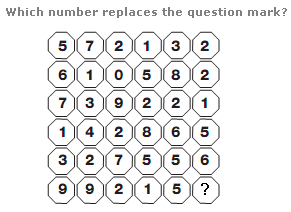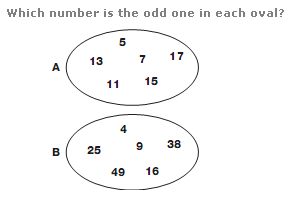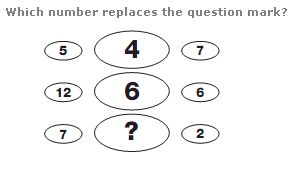# Puzzles - Number puzzles

### Exercise :: Number puzzlesAnswer : 4 Explanation : Working in rows from top to bottom, the sum of the digits in each row increases by 2 each time, from 20 to 30.Answer : A:15 B:38 Explanation : In the first oval, all numbers are Prime Numbers. In the second oval, all numbers are Square Numbers.Answer : 3 Explanation : Working in rows, the number in the central oval equals the sum of the left and right hand numbers, divided by 3.